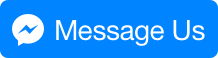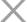Industry News

# Bending Basics: 6 steps to successful die selection for press brakes

Views : 586
Update time : 2017-02-15 13:59:43

# Bending Basics: 6 steps to successful die selection for press brakes

## Choosing the right die for an application hinges on the tooling and press brakes you have, and the tonnage limitations of both.1. Ensure that the specified inside bend radius is not less than the Min. sharp bend radius. If it isn’t, the inside bend radius can’t be achieved physically, short of stamping or bottoming. That’s because, when the bend turns sharp, the punch starts to dig a ditch into the material. For mild steel, a bend usually turns sharp when the inside radius reaches about 63 percent of material thickness. In your case, of course, you’re looking to achieve a ratio of 1-to-1 for material thickness to inside bend radius, which is certainly achievable, as long as your tooling and machines can handle the tonnage requirements.

2. Select the die opening. When it comes to any kind of machine, you generally do not want to overuse or underuse it. You get the most out of the machine at half of the maximum working value. That being said, isn’t the combination of the die, punch, and material really a “machine”? Of course it is. So what is half the working value of a die? Under perfect conditions, that point occurs halfway down the die face, as shown in Figure 1.

To find the geometrically perfect die opening—one in which the bend occurs halfway down the die face—use the following formula: (Outside bend radius × 0.7071) × Factor = Perfect die opening.

The factor in the formula is a multiplier, and a multiplier of 4.0 would give you a value as close to geometrically perfect as possible, practically speaking, but with no allowance for springback. To account for springback, increase the multiplier slightly. In material thicknesses less than 0.125 in., a realistic working multiplier is 4.85. In material between 0.125 and 0.250 in., the multiplier is 5.85 in. (material more than 0.250 in. thick is calculated differently). This die selection method keeps the relationships consistent whether the radius is large and the material thin, or the material is thick and the radius small.

In your situation, you would calculate the following: (Outside bend radius × 0.7071) × Factor = Perfect die opening; or (0.150 in. × 0.7071) × 4.85 = 0.514 in. Of course, your shop probably doesn’t have a die-opening width of 0.514 in., so you’ll likely need to choose the closest width available, between a 0.472- in. or a 0.551 in. die. Choosing the closest available die opening will keep your inside bend radius as close as possible to the called value. This assumes that excess tonnage is not an issue if a smaller die is used.

(Note that using a factor of 4.0, the die width value would be 0.424 in., which, at least in the theoretical sense, is geometrically perfect for the job, but again does not take springback into consideration.)

3. Calculate the tonnage requirements. Now that you’ve determined the ideal die opening, you need to make sure it doesn’t exceed available tonnage of your press or tooling. To calculate this, use the following: [(575 × Material thickness2)/Die opening] × Material factor =Tonnage per foot.

We use the 60,000-PSI-tensile AISI 1035 (the most common type of cold-rolled steel used) as a baseline and so give it a material factor value of 1. To obtain a factor for a specific material, you can perform a simple comparison of tensile strengths, working with 60,000-PSI tensile as the baseline. If your 304 stainless is specified as having 85,000-PSI tensile, then you divide that tensile by 60,000 to get 1.4. So your tonnage calculation would be: [(575 × 0.005625) / 0.551] × 1.4 = 8.22 tons per foot. You will need to consider the length of the bend as well. If you’re within the tonnage limits of your tooling and press brake, then it’s on to the next step.

4. If the die width is acceptable, calculate the bend radius using the 20 percent rule. Start with the median value. Back to our 304 stainless example, the median percentage is 21. Multiply this percentage by the actual die opening you’ll be using, and you get the resulting inside bend radius: 0.551 in. × 0.21 = 0.1157-in. inside bend radius.

The actual radius will be approximately 0.116 in., which is as close to 1-to-1 as you can get in an air form. Yes, the radius is larger than a 1-to-1 ratio of bend radius to material thickness, but the die also is larger than perfect. Even a geometrically perfect die width would yield an inside radius slightly larger than the material thickness. Short of stamping, an exact 1-to-1 ratio is not possible without custom tooling.

5. Use this inside bend radius value to calculate the bend deduction. You now insert this inside bend radius value into your bend deduction formulas. Software has automated these calculations nowadays, but for a review of the math, check out “How the inside bend radius forms,” available at www.thefabricator.com.

6. Use the select tool set to achieve the calculated bend deduction. You’ve determined that the inside bend radius is physically possible; you’ve select a die-opening width that will get you as close as possible to the desired inside bend radius; you calculated your bend deductions based on the 20 percent rule; and you’ve taken available tonnage and springback into account. Considering all this, you’re well on your way to building perfect parts.